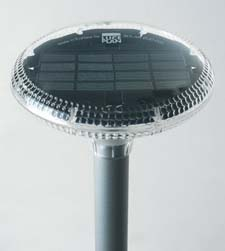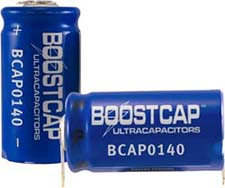HOME Hobby Corner Dave's Circuits Electronic Resources Book Corner Contact Info

 Back of the Envelope Previous Issues

Long Life Solar Powered LED Light

I got a call from a lighting company a while back.  They had a request from a construction company for an unusual solar powered LED light, which that was to be used on a new office building.  The LED light was to be mounted permanently into some fixtures on either side of some steps leading up the front door.The company had considered some off the shelf commercial solar powered pathway lights but they wanted the light to last much longer than possible with conventional rechargeable batteries.  They wanted something, which would last 10 or more years.  Would it be practical to use a supercapacitor instead of a battery? The company said that after some experiments they determined that the light from a white LED being driven at 10ma emitted sufficient light for their application.  They said, they wanted enough energy storage for 16 hours of dark conditions. Using this information as a starting point, let’s see how big the capacitor would have to be.
LED Energy Required:  A typical white LED has a forward voltage of 3.2v to 3.6v.  Let’s use the higher figure.  The company said that the LED needed 10ma of current for 16 hours.  So, the total energy pumped into the LED is: 3.6v X 0.01A X 16 hours x 3600 sec/hr = 2,074 joules.
Supercapacitor Energy Storage:  Unlike batteries, the voltage across a super capacitor drops as energy is drawn from it at a predictable rate.  But, not all the energy stored in the capacitor can be used.  A reasonable figure for the energy left in a supercapacitor when it approaches a fully discharged point is 10%. Thus, 90% of the available energy could be made available to drive the LED. So, if we need roughly 2000 joules to run the LED, we should plan on at least 2,200 joules of stored energy in the capacitor.  But, this figure does not include additional losses.
The most efficient way to convert the voltage across the capacitor into a constant current for the LED is with a DC to DC converter circuit.  These circuits are typically 80% or more efficient.  Let’s use the 80% figure. If we start with 2200 joules, then we will need an extra 20% to make up for the losses in the converter circuit.  That brings the total up to 2640 joules of needed storage.  Let’s round this off to 3,000 joules.
Most DC to DC converters are “buck” type regulators so they require a minimum voltage higher than the 3.6v output needed to run the LED.  I estimate that a capacitor voltage of at least 4.6v is needed to run the converter.  Let’s round this off to 5v.  So, at the end of the discharge cycle, 5v would be left in the capacitor, which we have defined as the 10% level. The energy stored in a capacitor is defined as: E = ˝ CV^2.  E is the energy in joules, C is the capacitance in farads and V is the voltage.   If we are leaving 10% of 3000 joules in the capacitor at a voltage of 5v, then the capacitance calculated to be: 24 farads.
Using the same energy equation as above, when the capacitor is fully charged to the 3000 joule level, the voltage V would be about 16 volts. So, the fully charged capacitor would start out at 16v, storing 3000 joules and be discharged down to 5v in about 16 hours of LED operation.
Most super capacitors have a voltage rating between 2.5v and 2.7v rating.  To achieve a total capacitance of 24 farads with 16 volts, six 144 farad capacitors with a 2.7v rating, would need to be wired in series.
 Maxwell 140 Farad 2.7v Capacitor:  Maxwell makes a 140 farad part (part number BCAP0140) which measures one inch in diameter and two inches long. A bank of 6 such devices would require a 3 inch wide, 2 inch deep and 2 inch high volume for a total of 12 cubic inches.  Even in large quantities these parts will cost about \$10 each for a total cost of \$60 for 6 devices.  In addition, some electronics would be needed to balance the voltage across these 6 parts, during charging.  In this 6 pack configuration, when fully charged, the supercapactor would store 3,000 joules in a pack volume of 12 cubic inches.  This is a density of 250 joules per cubic inch.  In comparison, a battery pack made of four 0.8amp-hour AAA NiMH
cells, would be very easy to charge and could power the single LED with little added electronics.  Such a pack would store 13,000 joules of energy. A AAA cell measures 0.4” in diameter and 1.75” long.  A pack of 4 such devices would yield a volume of 1.12 cubic inches for a density of 14,500 joules per cubic inch. This is 58 times denser than the supercapacitor and would only cost a few dollars. The only thing going in favor of the supercapacitor is its life expectancy of 10+ years.  So, although it is certainly possible to store energy in a supercapacitor to operate a LED light, it is not very cost effective and takes up much more room.  Only if the system must operate for more than 10 years does it become practical.
 February 2010       Issue 6 Page 1 Back Envelope Circuit Diagnosis Experimenter's Corner Good Idea gone Badly New Products Rants & Raves What the World needs Now Wily Widget